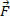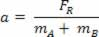Mechanics

# Blocks in horizontal movement

A and B , of masses A and B , supported on a horizontal friction surface and set in motion by virtue of the action of a horizontal force.

During the studies related to moving blocks, we must realize that when two or more blocks are subject to the action of the same force, remain in contact and are supported on a surface, they perform the same displacement in equal times. In this way, we can also say that they have the same velocity and also the same acceleration.

Analyzing the blocks separately, we can verify the forces that act on each of the blocks. See the figure below.

A and P B are the weight forces of blocks A and B ;
A and N B are the normal forces of blocks A and B ;
atA and F atB are the frictional forces on blocks A and B ;
F is the force applied to block A by an external agent;
AB and F BA are the forces of interaction between blocks A and B ( action and reaction ).

In this case, we can see that blocks A and B move only horizontally. Thus, we can say that horizontally, the resultant of the forces is zero ( R = 0 ). We also say that in each block the normal force balances the weight force. In module we have:

N = P = mg

Observing the blocks, if we verify that both are in motion, we should consider three situations:

– if the force F is greater than the sum of the friction forces, we say that the blocks are in accelerated motion with equal acceleration for both. In this case, the resultant force on the system will be given by:

R = F – (F at A + F at B )

– if the force F is equal to the sum of the intensities of the friction forces, the blocks will be in uniform motion with the same speed.

– if the horizontal surface is considered smooth, the intensity of the resultant force will be given by:

R = F

Therefore, to calculate the common acceleration of the two blocks just use the following equation:

R =m .a
R =(m A + m B ) .az